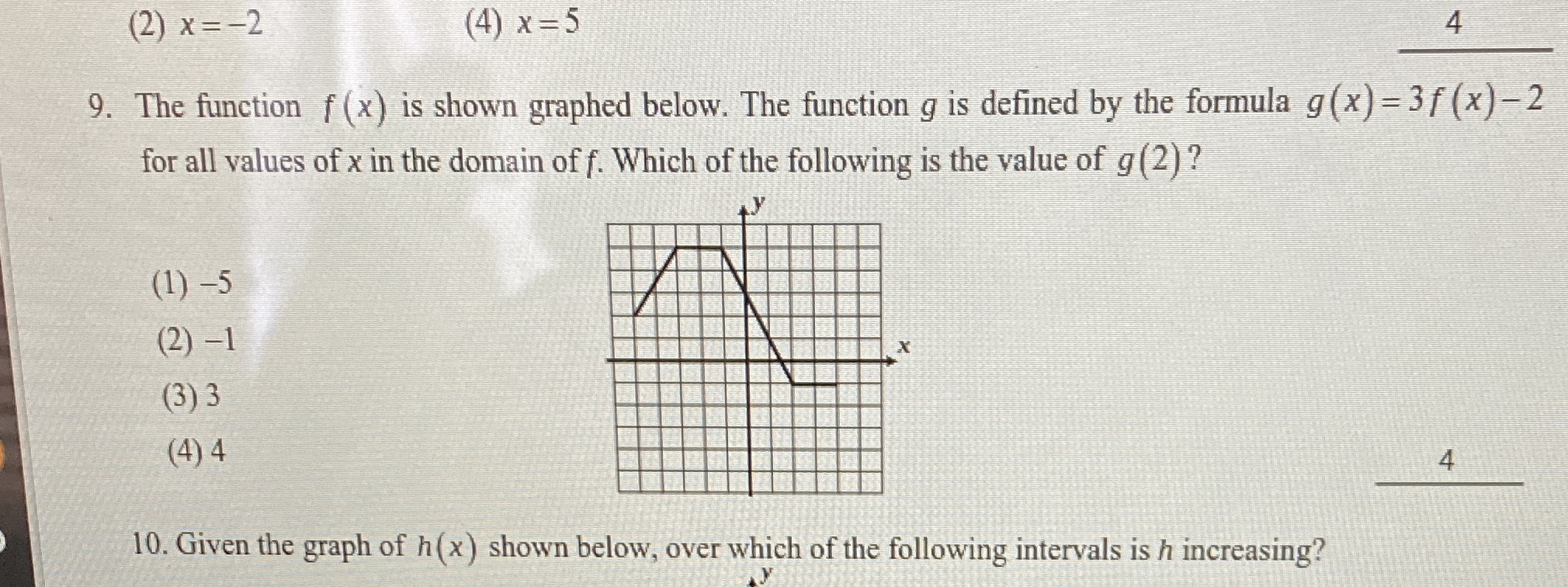### ¿Todavía tienes preguntas de matemáticas?

Pregunte a nuestros tutores expertos
Algebra
PreguntaThe function $$f ( x )$$ is shown graphed below. The function $$g$$ is defined by the formula $$g ( x ) = 3 f ( x ) - 2$$ for all values of $$x$$ in the domain of $$f$$ . Which of the following is the value of $$g ( 2 )$$ ?

(1)$$- 5$$

(2)$$- 1$$

(3) $$3$$

(4) $$4$$